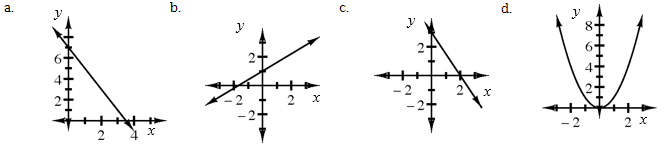Home > CCA2 > Chapter 1 > Lesson 1.1.1 > Problem1-8

1-8.

The graph for part (d) of problem 1-7 is different from the other three graphs. Homework Help ✎1. Explain how the graph is different from the other three graphs.

What do the other three graphs have in common?

2. What in the equation of part (d) makes its graph different?

What is different about $x$?

3. What is the graph of part (d) called?

A parabola.